# OpenMP Examples

##### Experimental html version of Parallel Programming in MPI, OpenMP, and PETSc by Victor Eijkhout. download the textbook at https:/theartofhpc.com/pcse
$\newcommand\inv{^{-1}}\newcommand\invt{^{-t}} \newcommand\bbP{\mathbb{P}} \newcommand\bbR{\mathbb{R}} \newcommand\defined{ \mathrel{\lower 5pt \hbox{{\equiv\atop\mathrm{D}}}}}$ 30.1 : N-body problems
30.1.1 : Solution 1: no conflicting writes
30.1.2 : Solution 2: using atomics
30.1.3 : Solution 3: all interactions atomic
30.2 : Tree traversal
30.3 : Depth-first search
30.4 : Filtering array elements
30.4.1 : Attempt 1: reduction
30.4.2 : Attempt 2: sequential appending
30.4.3 : Attempt 3: using tasks

# 30 OpenMP Examples

## 30.1 N-body problems

crumb trail: > omp-examples > N-body problems

So-called N-body problem s come up with we describe the interactions between a, probably large, number of entities under a force such as gravity. Examples are molecular dynamics and star clusters.

While clever algorithms exist that take into account the decay of the force over distance, we here consider the naive algorithm that explicitly computes all interactions.

A particle has $x,y$ coordinates and a mass $c$. For two particles $(x_1,y_1,c_1)$, $(x_2,y_2,c_2)$ the force on particle 1 from particle 2 is: \begin{equation} \overrightarrow F_{12} = \frac{c_1\cdot c_2}{\sqrt{ (x_2-x_1)^2+(y_2-y_1)^2 }} \cdot \overrightarrow r_{12} \end{equation} where $\overrightarrow r_{12}$ is the unit vector pointing from particle 2 to 1. With $n$ particles, each particle $i$ feels a force \begin{equation} \overrightarrow F_i = \sum_{j\not=i} \overrightarrow F_{ij}. \end{equation}

// molecularstruct.c
struct point{ double x,y; double c; };
struct force{ double x,y; double f; };

/* Force on p1 from p2 */
struct force force_calc( struct point p1,struct point p2 ) {
double dx = p2.x - p1.x, dy = p2.y - p1.y;
double  f = p1.c * p2.c / sqrt( dx*dx + dy*dy );
struct force exert = {dx,dy,f};
return exert;
}

Force accumulation:
void add_force( struct force *f,struct force g ) {
f->x += g.x; f->y += g.y; f->f += g.f;
}
void sub_force( struct force *f,struct force g ) {
f->x -= g.x; f->y -= g.y; f->f += g.f;
}


In C++ we can have a class with an addition operator and such:

// molecular.cxx
class force {
private:
double _x{0.},_y{0.}; double _f{0.};
public:
force() {};
force(double x,double y,double f)
: _x(x),_y(y),_f(f) {};

force operator+( const force& g ) {
return { _x+g._x, _y+g._y, _f+g._f };
}


For reference, this is the sequential code:

for (int ip=0; ip<N; ip++) {
for (int jp=ip+1; jp<N; jp++) {
struct force f = force_calc(points[ip],points[jp]);
sub_force( forces+jp,f );
}
}

Here $\overrightarrow F_{ij}$ is only computed for $j>i$, and then added to both $\overrightarrow F_i$ and $\overrightarrow F_j$.

In C++ we use the overloaded operators:

for (int ip=0; ip<N; ip++) {
for (int jp=ip+1; jp<N; jp++) {
force f = points[ip].force_calc(points[jp]);
forces[ip] += f;
forces[jp] -= f;
}
}


Exercise Argue that both the outer loop and the inner are not directly parallelizable.
End of exercise

We will now explore a number of different strategies for parallelization. All tests are done on the TACC Frontera cluster, which has dual-socket Intel Cascade Lake nodes, with a total of 56 cores. Our code uses 10 thousand particles, and each interaction evaluation is repeated 10 times to eliminate cache loading effects.

### 30.1.1 Solution 1: no conflicting writes

crumb trail: > omp-examples > N-body problems > Solution 1: no conflicting writes

In our first attempt at an efficient parallel code, we compute the full $N^2$ interactions. One solution would be to compute the $\overrightarrow F_{ij}$ interactions for all $i,j$, so that there are no conflicting writes.

for (int ip=0; ip<N; ip++) {
struct force sumforce;
sumforce.x=0.; sumforce.y=0.; sumforce.f=0.;
#pragma omp parallel for reduction(+:sumforce)
for (int jp=0; jp<N; jp++) {
if (ip==jp) continue;
struct force f = force_calc(points[ip],points[jp]);
sumforce.x += f.x; sumforce.y += f.y; sumforce.f += f.f;
} // end parallel jp loop
} // end ip loop


In C++ we use the fact that we can reduce on any class that has an addition operator:

for (int ip=0; ip<N; ip++) {
force sumforce;
#pragma omp parallel for reduction(+:sumforce)
for (int jp=0; jp<N; jp++) {
if (ip==jp) continue;
force f = points[ip].force_calc(points[jp]);
sumforce += f;
} // end parallel jp loop
forces[ip] += sumforce;
} // end ip loop


This increases the scalar work by a factor of two, but surprisingly, on a single thread the run time improves: we measure a speedup of 6.51 over the supposedly optimal' code.

Exercise What would be an explanation?
End of exercise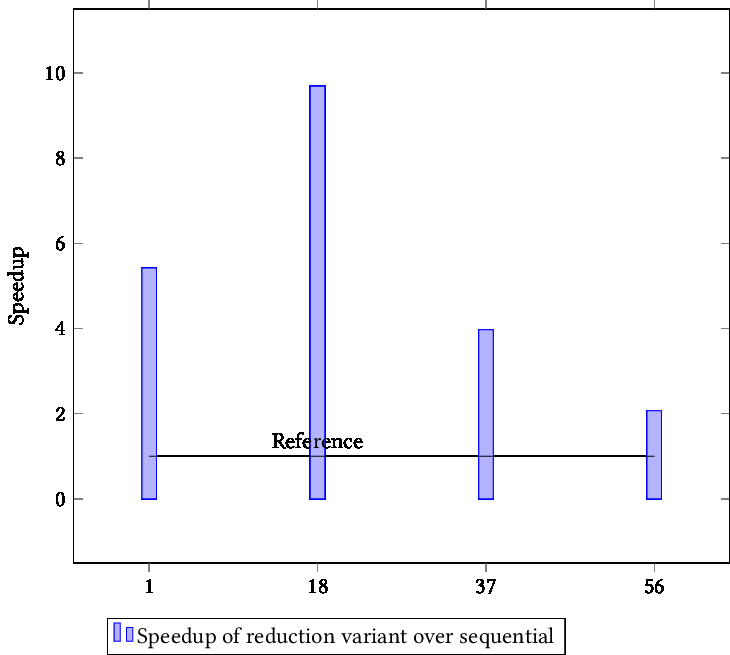TIKZPICTURE 30.1: {Speedup of reduction variant over sequential}

However, increasing the number of threads has limited benefits for this strategy. Figure  30.1 shows that the speedup is not only sublinear: it actually decreases with increasing core count.

Exercise What would be an explanation?
End of exercise

### 30.1.2 Solution 2: using atomics

crumb trail: > omp-examples > N-body problems > Solution 2: using atomics

Next we try to parallelize the outer loop.

#pragma omp parallel for schedule(guided,4)
for (int ip=0; ip<N; ip++) {
for (int jp=ip+1; jp<N; jp++) {
struct force f = force_calc(points[ip],points[jp]);
sub_force( forces+jp,f );
}
}

To deal with the conflicting jp writes, we make the writes atomic:
void sub_force( struct force *f,struct force g ) {
#pragma omp atomic
f->x -= g.x;
#pragma omp atomic
f->y -= g.y;
#pragma omp atomic
f->f += g.f;
}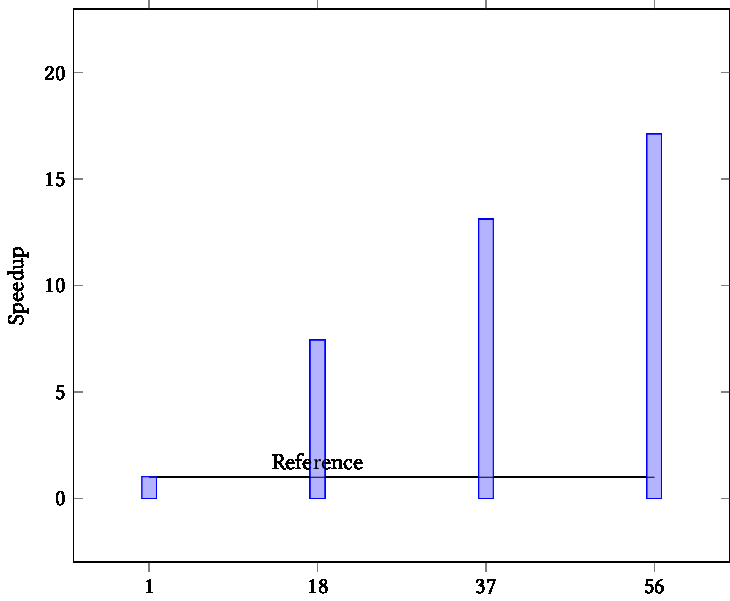FIGURE 30.2: Speedup of triangular loop with atomic update

This works fairly well, as figure  30.1.2 shows.

### 30.1.3 Solution 3: all interactions atomic

crumb trail: > omp-examples > N-body problems > Solution 3: all interactions atomic

But if we decide to use atomic updates, we can take the full square loop, collapse the two loops, and make every write atomic.

#pragma omp parallel for collapse(2)
for (int ip=0; ip<N; ip++) {
for (int jp=0; jp<N; jp++) {
if (ip==jp) continue;
struct force f = force_calc(points[ip],points[jp]);
} // end parallel jp loop
} // end ip loop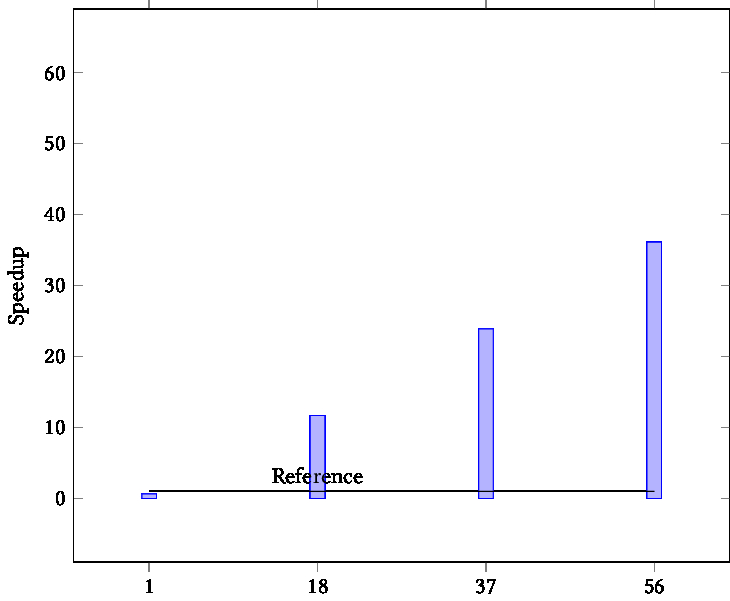TIKZPICTURE 30.3: Speedup of atomic full interaction calculation

Figure  30.3 shows that this is pretty close to perfect.

Everything in one plot in figure  30.4  .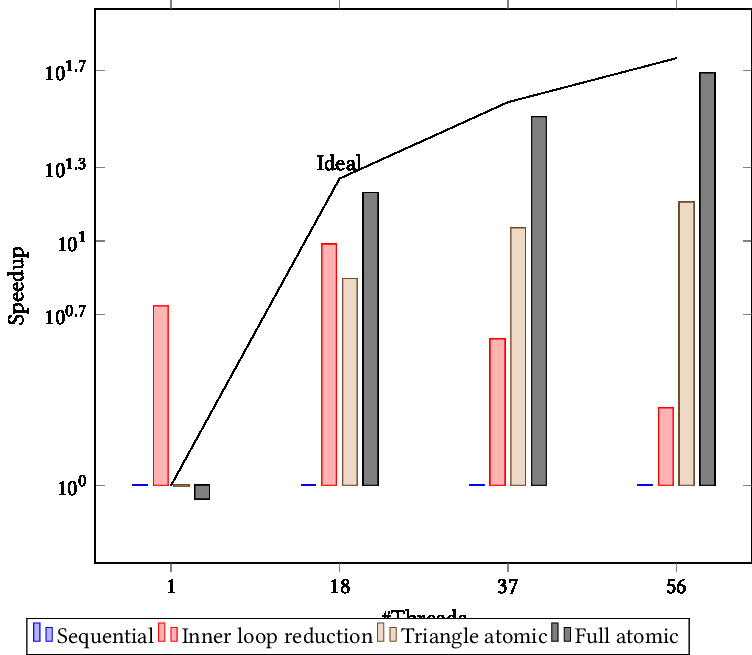TIKZPICTURE 30.4: All strategies together

## 30.2 Tree traversal

crumb trail: > omp-examples > Tree traversal

OpenMP tasks are a great way of handling trees.

In post-order tree traversal you visit the subtrees before visiting the root. This is the traversal that you use to find summary information about a tree, for instance the sum of all nodes, and the sums of nodes of all subtrees:

\begin{displayalgorithm} \For{all children $c$} {compute the sum $s_c$}\; $s \leftarrow \sum_c s_c$ \end{displayalgorithm}

Another example is matrix factorization: \begin{equation} S = A_{33} - A_{31}A_{11}\inv A_{13} - A_{32}A_{22}\inv A_{23} \end{equation} where the two inverses $A_{11}\inv,A_{22}\inv$ can be computed independently and recursively.

## 30.3 Depth-first search

crumb trail: > omp-examples > Depth-first search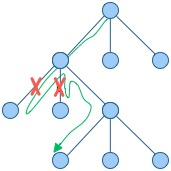In this section we look at the eight queens' problem, as an example of DFS : is it possible to put eight queens on a chess board so that none of them threaten each other? With DFS  , the search space of possibilities is organized as a tree -- each partial solution leads to several possibilities for the next steps -- which is traversed in a particular manner: a chain of possibilities is extended as far as feasible, after which the search backtracks to the next chain.

The sequential implementation is easy enough. The main program fires off:

placement initial; initial.fill(empty);
auto solution = place_queen(0,initial);

where I hope you can take the details on trust.

The recursive call then has this structure:

optional<placement> place_queen(int iqueen,const placement& current) {
for (int col=0; col<N; col++) {
placement next = current;
next.at(iqueen) = col;
if (feasible(next)) {
if (iqueen==N-1)
return next;
auto attempt = place_queen(iqueen+1,next);
if (attempt.has_value())
return attempt;
} // end if(feasible)
}
return {};
};

(This uses the C++17 optional header.) At each iqueen level we
• go through a loop of all column positions;
• filter out positions that are not feasible;
• report success if this was the last level; or
• recursively continue the next level otherwise.

This problem seems a prime candidate for OpenMP tasks, so we start with the usual idiom for the main program:

placement initial; initial.fill(empty);
optional<placement> eightqueens;
#pragma omp parallel
#pragma omp single
eightqueens = place_queen(0,initial);


We create a task for each column, and since they are in a loop we use taskgroup rather than taskwait  .

#pragma omp taskgroup
for (int col=0; col<N; col++) {
placement next = current;
next.at(iqueen) = col;
if (feasible(next)) {
// stuff
} // end if(feasible)
}


However, the sequential program had return and break statements in the loop, which is not allowed in workshare constructs such as taskgroup  . Therefore we introduce a return variable, declared as shared:

// queens0.cxx
optional<placement> result = {};
for (int col=0; col<N; col++) {
placement next = current;
next.at(iqueen) = col;
if (feasible(next)) {
if (iqueen==N-1) {
result = next;
} else { // do next level
auto attempt = place_queen(iqueen+1,next);
if (attempt.has_value()) {
result = attempt;
}
} // end if(iqueen==N-1)
} // end if(feasible)
}
return result;


So that was easy, this computes the right solution, and it uses OpenMP tasks. Done?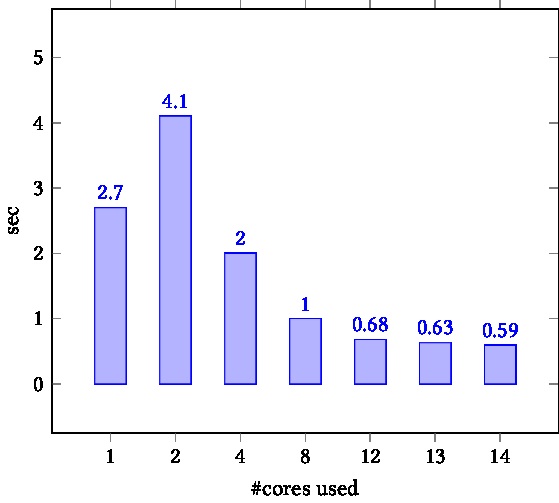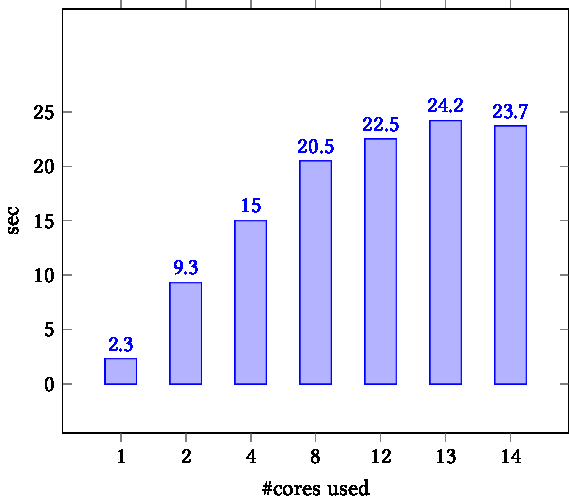TIKZPICTURE 30.5: Using taskgroups for $N=12$; left Intel compiler, right GCC

Actually this runs very slowly because, now that we've dispensed with all early breaks from the loop, we in effect traverse the whole search tree. (It's not quite breadth-first, though.) Figure  30.5 shows this for $N=12$ with the Intel compiler (version 2019) in the left panel, and the GNU compiler (version 9.1) in the middle. In both cases, the blue bars give the result for the code with only the taskgroup directive, with time plotted as function of core count.

We see that, for the Intel compiler, running time indeed goes down with core count. So, while we compute too much (the whole search space), at least parallelization helps. With a number of threads greater than the problem size, the benefit of parallelization disappears, which makes some sort of sense.

We also see that the GCC compiler is really bad at OpenMP tasks: the running time actually increases with the number of threads.

Fortunately, with OpenMP- we can break out of the loop with a cancel of the task group:

// queenfinal.cxx
if (feasible(next)) {
if (iqueen==N-1) {
result = next;
} else { // do next level
auto attempt = place_queen(iqueen+1,next);
if (attempt.has_value()) {
result = attempt;
}
} // end if (iqueen==N-1)
} // end if (feasible)


Surprisingly, this does not immediately give a performance improvement. The reason for this is that cancellation is disabled by default, and we have to set the environment variable

OMP_CANCELLATION=true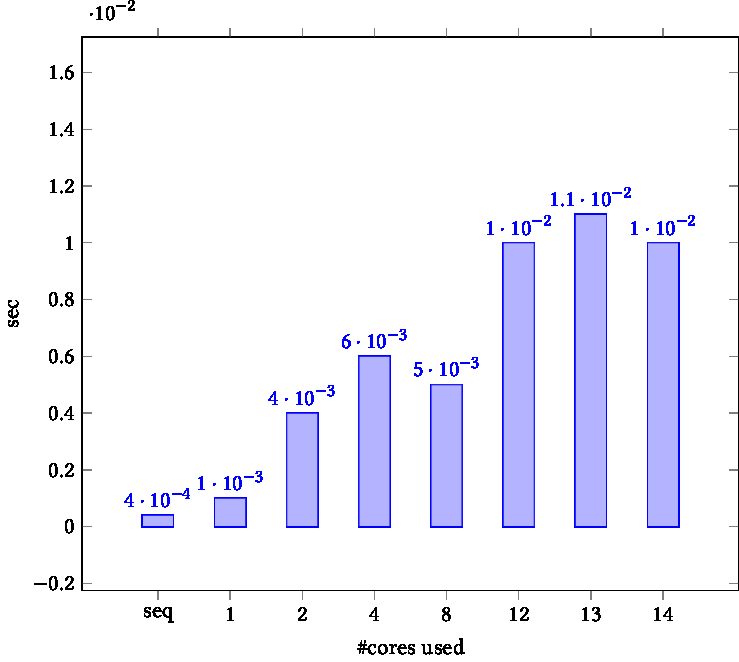TIKZPICTURE 30.6: Using taskgroup cancelling, Intel compiler

With that, we get very good performance, as figure  30.6 shows, which lists sequential time, and multicore running time on the code with cancel directives. Running time is now approximately the same as the sequential time. Some questions are still left:

• Why does the time go up with core count?
• Why is the multicore code slower than the sequential code, and would the parallel code be faster than sequential if the amount of scalar work (for instance in the feasible function) would be larger?

One observation not reported here is that the GNU compiler has basically the same running time with and without cancellation. This is again shows that the GNU compiler is really bad at OpenMP tasks.

## 30.4 Filtering array elements

crumb trail: > omp-examples > Filtering array elements

Let's assume we have an array of (integer, for the sake of the argument) elements and we want to construct a subarray of only those elements that satisfy some test

bool f(int);


C++ note We will do this example only in C++ because of its ease of handling std::vector s. End of C++ note

The sequential code is as follows:

vector<int> data(100);
// fil the data
vector<int> filtered;
for ( auto e : data ) {
if ( f(e) )
filtered.push_back(e);
}

There are two problems here. First is the race condition on the filtered array. Even if we fix this with a critical region, there remains the lack of ordering of inserted elements.

The key to the solution is to let each thread have a local array, and then to concatenate these:

#pragma omp parallel
{
vector<int> local;
# pragma omp for
for ( auto e : data )
if ( f(e) )
local.push_back(e);
filtered += local;
}

where we have used an append operation on vectors:
// filterreduct.cxx
template<typename T>
vector<T>& operator+=( vector<T>& me, const vector<T>& other ) {
me.insert( me.end(),other.begin(),other.end() );
return me;
};


### 30.4.1 Attempt 1: reduction

crumb trail: > omp-examples > Filtering array elements > Attempt 1: reduction

We could use the plus-is operation to declare a reduction:

#pragma omp declare reduction\
(	\
+:vector<int>:omp_out += omp_in	\
) \
initializer( omp_priv = vector<int>{} )


The problem here is that OpenMP reductions can not be declared non-commutative, so the contributions from the threads may not appear in order.

\cxxsnippetwithoutput{cppvectordoreduct}{code/omp/cxx}{filterreduct}

### 30.4.2 Attempt 2: sequential appending

crumb trail: > omp-examples > Filtering array elements > Attempt 2: sequential appending

If we don't use a reduction, but do the appending explicitly, we first of all have to make this operation into a critical section. Secondly, we have to impose the correct ordering.

Here is an attempt to do this by keeping a shared counter: \cxxsnippetwithoutput{cppvectordoseq}{code/omp/cxx}{filteratomic}

The problem here is that threads who decide it's not their turn will simply skip the append operation: there is no way to tell them to wait their turn. (You could experiment with a while loop. Try it.)

The best solution is to use a completely different mechanism.

### 30.4.3 Attempt 3: using tasks

crumb trail: > omp-examples > Filtering array elements > Attempt 3: using tasks

crumb trail: > omp-examples > Thread synchronization

Let's do a producer-consumer model\footnote{This example is from Intel's excellent OMP course by Tim Mattson}. This can be implemented with sections, where one section, the producer, sets a flag when data is available, and the other, the consumer, waits until the flag is set.

#pragma omp parallel sections
{
// the producer
#pragma omp section
{
... do some producing work ...
flag = 1;
}
// the consumer
#pragma omp section
{
while (flag==0) { }
... do some consuming work ...
}
}

One reason this doesn't work, is that the compiler will see that the flag is never used in the producing section, and that is never changed in the consuming section, so it may optimize these statements, to the point of optimizing them away.

The producer then needs to do:

 ... do some producing work ...
#pragma omp flush
#pragma atomic write
flag = 1;
#pragma omp flush(flag)

and the consumer does:
#pragma omp flush(flag)
while (flag==0) {
#pragma omp flush(flag)
}
#pragma omp flush

This code strictly speaking has a race condition on the flag variable.

The solution is to make this an atomic operation and use an atomic pragma here: the producer has

#pragma atomic write
flag = 1;

and the consumer:
while (1) {
#pragma omp flush(flag)